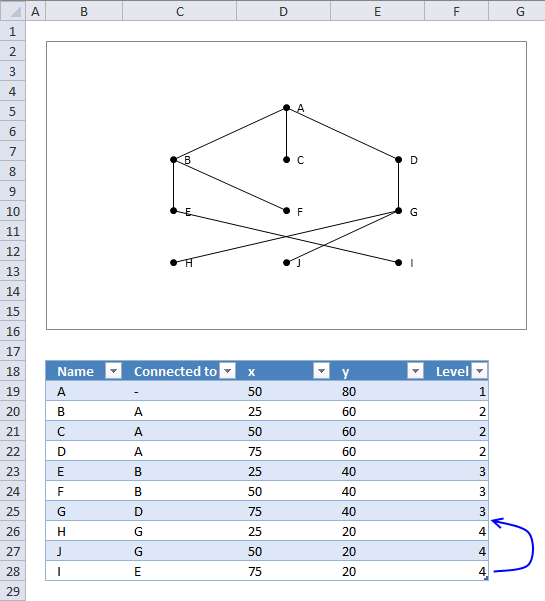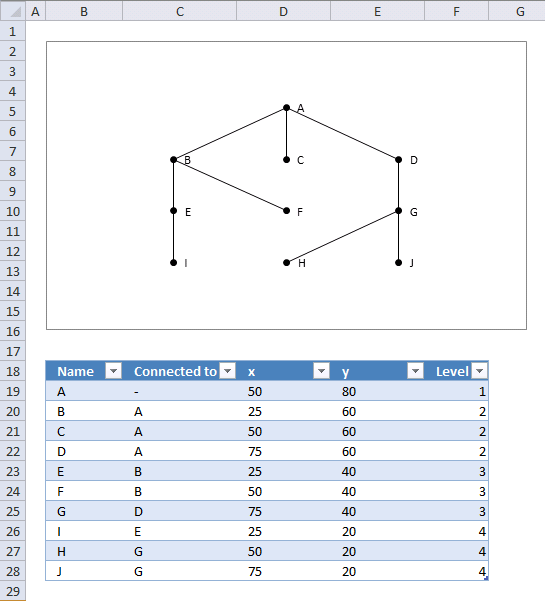Author: Oscar Cronquist Article last updated on June 28, 2013

The macro in this example adds series to a scatter chart. The first series is the dots and their names. The remaining series are the lines connecting the dots. They originate from the "Connected to" column in the table below the chart.

The table calculates the x and y chart coordinates using the "x", "y" and "Levels" columns.

What can you do with this macro?

• Hierarchical charts
• Organization charts
• Parent - child relationships
=(100/(COUNTIF([Level],[@Level])+1))*COUNTIF(\$F\$19:F19,[@Level])

Array formula in cell E19:

=100/(MAX([Level])+1)*(MAX([Level])-[@Level]+1)

Formula in cell F19:

=IF(C19="-",1,INDEX([Level],MATCH(C19,[Name],0))+1)

You can´t connect a "child" to two or more "parents", but you can connect two or more "children" to a "parent".

VBA Code

Dim i As Single, rc As Single, r As Single
'Remove previous series
rc = ActiveSheet.ChartObjects("Chart 1").Chart.SeriesCollection.Count
For i = 1 To rc
ActiveSheet.ChartObjects("Chart 1").Chart.SeriesCollection(1).Delete
Next i

With ActiveSheet.ChartObjects("Chart 1").Chart.SeriesCollection.NewSeries
.Values = ActiveSheet.Range("Table1[y]")
.XValues = ActiveSheet.Range("Table1[x]")
.MarkerStyle = 8
.MarkerSize = 5
.Format.Fill.Solid
.Format.Fill.ForeColor.ObjectThemeColor = msoThemeColorText1
.Format.Line.Visible = msoFalse

rc = Range("Table1[Name]").Rows.Count
For r = 1 To rc
.Points(r).ApplyDataLabels
.Points(r).DataLabel.Text = Range("Table1[Name]").Cells(r).Value
Next r
End With

On Error Resume Next
rc = Range("Table1[Name]").Rows.Count
For i = 2 To rc
r = Application.WorksheetFunction.Match(Range("Table1[Connected to]").Cells(i).Value, Range("Table1[Name]"), 0)
With ActiveSheet.ChartObjects("Chart 1").Chart.SeriesCollection.NewSeries
.Values = Array(Range("Table1[y]").Cells(i), Range("Table1[y]").Cells(r))
.XValues = Array(Range("Table1[x]").Cells(i), Range("Table1[x]").Cells(r))
.MarkerStyle = -4142
.Format.Line.ForeColor.ObjectThemeColor = msoThemeColorText1
.Format.Line.Weight = 1
End With
Next i
On Error GoTo 0
End Sub

If you are interested in how to work with charts using vba, I recommend reading John Peltier´s post:
VBA Code to Add Chart Objects and Series